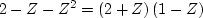Next: Convolution equation and program Up: SAMPLED DATA AND Z-TRANSFORMS Previous: Convolution with Z-transform

## Dissecting systems by factoring

Consider a system with an impulse response (2,-1,-1). Its Z-transform is B(Z) = 2 - Z - Z2. This polynomial can be factored into.Thus our original filter could be thought of as a cascade of two filters, (2,1) and (1,-1). Either of the two filters could be applied first and the other second: the output would be the same. Since any polynomial can be factored,  any impulse response can be simulated by a cascade of two-term filters (impulse responses whose Z-transforms are linear in Z).Next: Convolution equation and program Up: SAMPLED DATA AND Z-TRANSFORMS Previous: Convolution with Z-transform
Stanford Exploration Project
10/21/1998